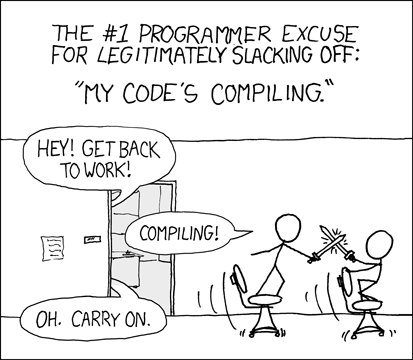- 3 mins

# Prime numbers.

Hopefully, by now, we know what they are. Do we, however, know an efficient way to add up every single one between 1 and 2,000,000?

This question, also known as problem 10 on Project Euler, seems pretty simple at face value. For beginners, the simplest and obvious solution is to solve it by brute force:

#### Exhibit A

This way would be fine if our upper limit was not two million. Running this code works, but it takes over 10 minutes to get the answer on my Acer Aspire S7-392 Ultrabook (a great computer, but it is not Watson).I am pretty sure Project Euler has a one minute compiling “rule” where you should get your results in under a minute, so this solution was obviously not acceptable.

The next thing I tried was putting the numbers in an ArrayList, from two to two million. Then, I ran them through a loop that deleted the numbers from the list if they were not prime. You can imagine this was not very efficient either - in fact, it was faster to brute force. After messing around with this (very messy) code for an extended period of time, I did some research on algorithms and stumbled upon the Sieve of Eratosthenes.

### Sieve of Eratosthenes

The Sieve of Eratosthenes works on the idea that, if number (n) is prime, any multiples of (n) will not be prime. So where n is prime, 2n would not be.

It took me a few tries to implement it correctly, but I believe I did it farily efficiently here:

#### Exhibit B

Instead of comparing each digit to every possible factor, we now only compare it to known prime numbers. Starting with 2, there are no digits in our array list of primes, so 2 is added to the prime list. The number 3 is compared to the array list of primes, which only consists of 2 - it cannot divide evenly, so 3 is added to the list of primes. When 4 is compared against the list of primes, it can divide evenly against 2, so it is not added to the list and the program breaks out since the number cannot be prime. And so on.

This, of course, makes our program take a fraction of the time to find a solution, because we are comparing each digit against a lot less numbers. When the upper limit is 2 million, the efficiency gains are much more noteworthy.

Time to find the solution in exhibit B: ~46 seconds.